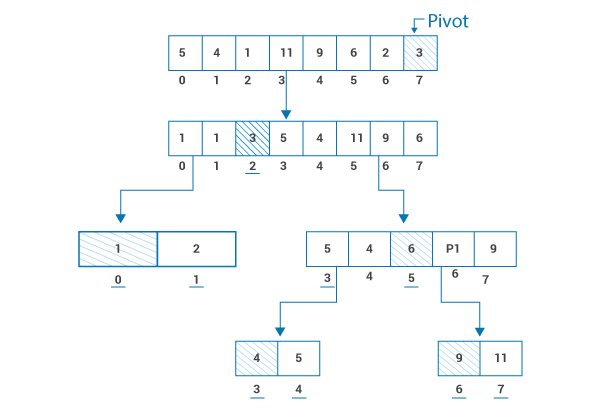# All you Need to Know About Quicksort in C++

Published on Sep 06,2019 24 Views### myMock Interview Service for Real Tech Jobs### myMock Interview Service for Real Tech Jobs

• Mock interview in latest tech domains i.e JAVA, AI, DEVOPS,etc
• Get interviewed by leading tech experts
• Real time assessment report and video recording

There are a plethora of sorting algorithms. Finding the right fit for your application is a task that requires a brief understanding of factors such as performance, time complexity, length of code, etc. of a particular algorithm. In this post, we will take a look at all the essential concepts required to implement the Quicksort in C++ in the following order:

## Understanding the Quicksort Algorithm

Just like Merge Sort, Quicksort follows the divide and conquer strategy. By using the divide and conquer strategy we divide the problem into many subproblems and solve them recursively. First, we will understand the whole process step by step and after that, with the help of an example, we will develop a deep understanding of the whole process.

1. First, we will ask for the unsorted array from the user.

2. Once we have our unsorted array we need to select a pivot value from the array. We can choose any value.

3. Once we select the pivot point after that we need to arrange the other elements of the array in such a way that, all the elements less than the pivot value should be placed to the right of the pivot value and all the elements greater than the pivot value are to be placed to the right of the pivot value.

4. We perform step 3 until we get our sorted array.

Now, let’s consider an example and implement the algorithm and see how it works.

Hello [5, 4, 1, 11, 9, 6, 2, 3] for this example we will always consider the pivot as the rightmost element of the list.Let’s go through each step and understand the logic that we used to solve the problem.

• First, we selected ‘3’ as our pivot and arranged all the elements less than ‘3’ in the right and all the elements greater than ‘3’ to the right.

• At this point, we have 2 subproblems. Let’s first solve the subproblem on the right. We selected one as our pivot and placed ‘2’ to the right.

• To solve the second subproblem we select ‘6’ as our pivot and place the elements as we discussed earlier.

• We have 2 more subproblems. The first one is solved by selecting 4 as the pivot and the second is solved by selecting 9 as the pivot. Finally, We have our sorted array with the elements placed at the underline index.

Note- The important point to understand here is that all the operations take place in the same array. New arrays are not created.

## Pseudocode for Quicksort in C++

```QuickSort(array[], start_index, end_index)
{
if (start_index < end_index)
{
partition_index = partition(array, start_index, end_index);

QuickSort(array, start_index, partition_index - 1);         QuickSort(array, partition_index + 1, end_index);
}
}```

## Program of Quicksort in C++

We understood the algorithm and developed a deep understanding of the working of the algorithm. Let’s implement Quicksort in C++ and write a program to sort an array.

```#include <iostream>

using namespace std;

void swap_elements(int* a, int* b)
{
int temp = *a;
*a = *b;
*b = temp;
}

int partition (int array[], int start_index, int end_index)
{
int pivot = array[end_index];
int i = (start_index - 1);

for (int j = start_index; j <= end_index- 1; j++)
{

if (array[j] <= pivot)
{
i++;
swap_elements(&array[i], &array[j]);
}
}
swap_elements(&array[i + 1], &array[end_index]);
return (i + 1);
}

void quickSort(int array[], int start_index, int end_index)
{
if (start_index < end_index)
{

int partition_index = partition(array, start_index, end_index);

quickSort(array, start_index, partition_index - 1);
quickSort(array, partition_index + 1, end_index);
}
}

void printArray(int array[], int number)
{
int i;
cout<<"Sorted Array: ";

for (i = 0; i < number; i++)
cout << array[i] << " ";
cout << endl;
}

int main()
{
int Hello;
int i;
int NumberofElements;
cout<<"Enter the number of elements to be sorted:"; cin>>NumberofElements;
cout<<"Enter the elements one by one: ";

for(i=0;i<NumberofElements;i++) { cin>>Hello[i];
}

quickSort(Hello, 0, NumberofElements-1);

printArray(Hello, NumberofElements);
return 0;
}```

Output:## Time Complexity

Let’s talk about the most important aspect of any sorting algorithm, i.e time complexity. It tells us about the performance of the algorithm in various scenarios. These values can help us in deciding if we can use this algorithm for our application.

• Best case- O(n)
• Average case- (nlogn)
• Worst-case- O(n2)

With this, we come to an end of this Quicksort in C++ article. If you wish to learn more, check out the Java Training by Edureka, a trusted online learning company. Edureka’s Java J2EE and SOA training and certification course is designed to train you for both core and advanced Java concepts along with various Java frameworks like Hibernate & Spring.

Got a question for us? Please mention it in the comments section of this blog and we will get back to you as soon as possible.